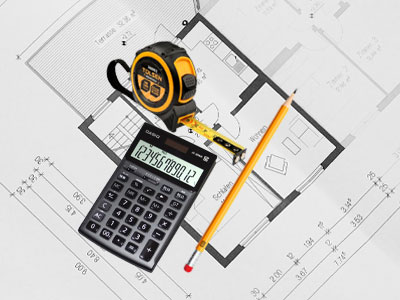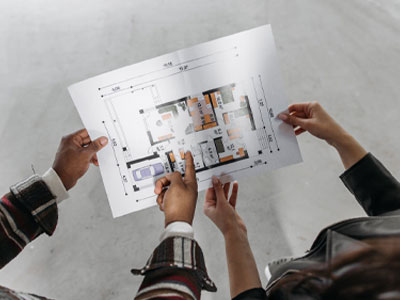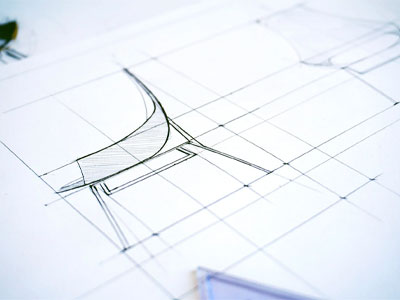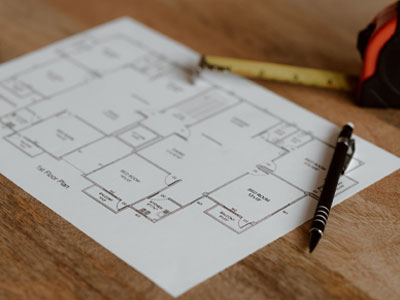# How to Calculate Square Footage of Your Own House

Planning to buy a house or sell one or want to renovate and place furniture requires measuring the square footage of your house in the first place. You might have mastered maths or various measurement skills, but calculating square footage could be trickier than you had thought.

Most of the people solely rely upon 3rd party’s stated measurements without ever doubting it. But this has to be taken very seriously as little difference in the measure could cost you a considerable amount of money.So it is better to be self-sufficient when calculating the square footage of your own house.

## Why Calculating Square Footage Important

The fact that Dhanmondi is practically self-contained, with no amenity destination more than a few steps away, is perhaps its best feature. In terms of getting around the city, numerous public buses run along with the Sat Masjid and Mirpur Road, linking Dhanmondi to Lalmatia, Mohammadpur, the New Market area, Agargaon, Shyamoli, Mirpur, and Kallyanpur.

Moreover, the Shahbag road connects the region to Malibagh, Kakrail, Motijheel, and Gulistan via public transportation, resulting in a mass network of connectedness that is almost incomparable to most of Dhaka's areas.### Why Calculating Square Footage Important

Calculating square footage is crucial for many reasons. When you plan to buy or sell a house, square footage is the only measurement criteria to determine its area.

So it’s easy to say how deep-rooted the square footage measurement is in terms of setting the price of a property. The square footage is also necessary to reevaluate the property’s worth and plan to renovate or organize the furniture.

In every step of the process, you will require the square footage calculation. Even in property tax, the amount would be as per the size of the property.### Equipment Required for Measuring Square Footage

The calculation process requires few materials of the following:

• Paper
• Pencil/pen
• Measuring tape
• Calculato

A smartphone or computer could replace paper, pencil, and calculator, but these things are advised for your best convenience.

### How to Calculate Square Footage of Your Own house

Calculating the square footage of your own house, room, or condo, you can apply the simple techniques mentioned below and get a proper measurement. If your place is rectangular, then the calculation would be straightforward. Follow the mentioned procedures for calculation:• Drawdown the shape of the house, which includes all the rooms similar to existing shapes. E.g., the drawing should look similar to afloor plan. Don’t worry; it’s just for a sketching purpose.
• Measure the length, width of each room, including the hallways and balconies. Try to take the feet count in the measurement tape.
• Now write down all the sizes that you measured in the corresponding rooms in the drawing. E.g., bedroom length: 15 feet, width: 20 feet.
• Now pull out the calculator, multiply the length and width of each room and write it on the drawing of the respective room. E.g., Size of the bedroom: 15 feet x 20 feet = 300 square feet.
• Add all the areas of the rooms together and get the total house measurement in square feet

The calculation here misses the area, including the walls of the rooms. So if you want a more precise calculation, try to include room walls in the calculation.

#### How to Calculate Square Footage of the House When Rooms are in Odd Shapes

The question must be peeping in your head that the equation seems about fine and easy for rectangular rooms and shapes. But what about circular, triangular, pentagonal, or hexagonal room sizes?

Well, there are solutions for them. The rooms in a house are mostly rectangular or square in shape. So the standard equation stays about the same. But if the room or space is in any shape other than a rectangle, we have to put it in that form’s equation. And pull out the total area measure.

To make the equation easier, try to break the room area into parts. The part resembles a rectangle, and the part resembles a triangle or circle, calculating the areas differently. This way, you can further reduce the complications• For Triangular room or space: (Height x Base)/2, for cases of a right-angle triangle. If the triangle is not right-angled, the equation will be different.
• For Circular room or space: Square of circle’s radius x pie. For a detailed calculation, look here.
• For pentagonal room or space: As pentagonal areas are a bit complicated to measure. Here is a detailed guide to help you with calculating the area of a pentagonal room or space.
• For hexagonal room or space: Almost similar to the pentagon, hexagon also requires a bit of struggle to bring down its exact area calculation. You can find the process broken down over here.

After calculation, the next step is simple and previously mentioned. Just add the room areas together and get the total square footage count.#### What is not included in Square Footage Calculation

In square footage measurement, stairway space, garage and basement are not included. So do not include these in the calculation of your house square footage.

You can also talk to the authority or previous owner regarding what is included or what is not in their count.

#### Conclusion

Differences in calculation could easily occur when different parties measure house square footage. The differences mostly happen because some parties measure the area by roof size, some include wall areas, some even measure the total space of the building. Ideal calculation keeps the room walls in the calculation.

The rightful authority must mention how the square footage was previously calculated. Now that you are well-equipped with all the measurement processes, never mind checking the house’s square footage before moving in or selling out a house.